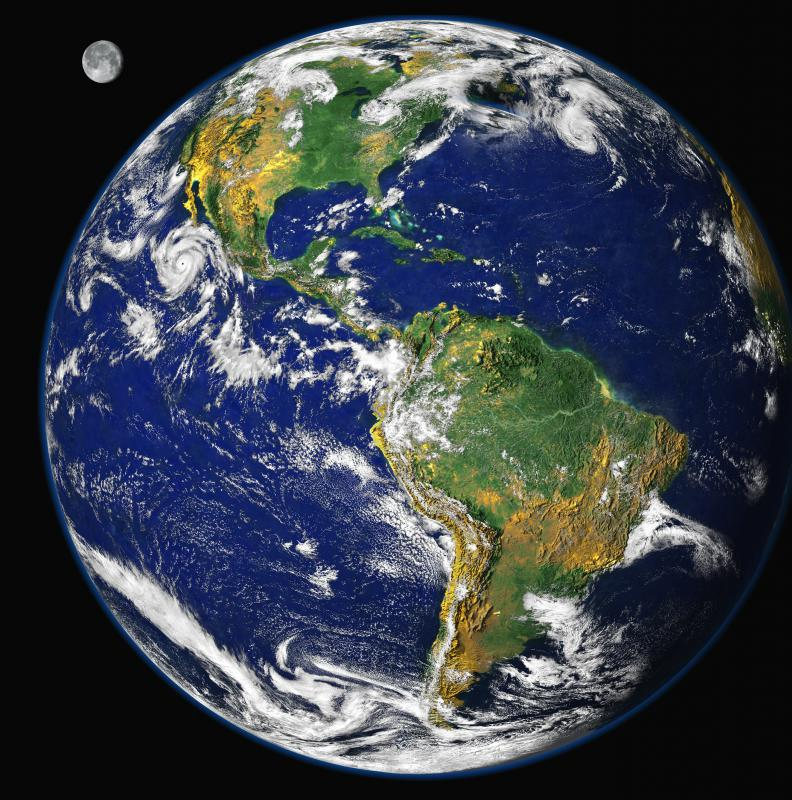# What is Acceleration of Gravity?

M.R. Anglin
M.R. AnglinAcceleration of gravity is the rate at which something plummets toward Earth's center.

All objects, no matter what the size, will fall at the same rate: the acceleration of gravity. This is the rate at which an object free-falls. That is, it is the rate at which an object accelerates toward the center of the Earth. This value is not a constant one but changes with the location of the free-falling object.

On Earth, the acceleration of gravity is approximately 32.2 ft/sec2 (9.8m/s2). This means that an object will accelerate 32.2ft/sec (9.8m/s) for every second it falls. In other words, the longer an object falls, the faster it is falling. Think of it as a car that is constantly accelerating. The car would keep going faster and faster the longer it was driven. Similarly, an object falling for three seconds will be going faster than an object falling for one second.

This acceleration rate depends largely on the surface the object is falling towards. Many of us will only experience gravity as it relates to Earth, but the number will change dramatically if we were on another celestial body. The acceleration of gravity is much less on the moon, for instance. In fact, it is a sixth that of Earth, a value of approximately 5.3 ft/s2 (1.6 m/s2). An object will fall toward the moon at a much slower rate.On the moon, the air is a vacuum, and so objects fall to the ground at the acceleration of the moon's gravity.

Using the equation, g=GM/R2, the acceleration of gravity of different objects in space can be calculated. In the equation, g is gravity, G is the gravitational constant, R is the radius of the planet, and M is the mass of the planet. Doing the calculations, physicists have determined that the acceleration of gravity on Jupiter is approximately 85.3ft/s2 (26m/s2). Pluto, on the other hand, has a value of 2 ft/s2 (0.61m/s2). You can see that planets with more mass have a greater acceleration of gravity than planets with less mass.All objects fall at the same rate, regardless of their size.

If the world was a vacuum, these values would represent real life. On the moon, the air is a vacuum, and so objects fall to the ground at the acceleration of the moon's gravity. On Earth, however, we have air resistance--the force of air pushing against an object as it falls. This is the reason that a feather floats down to Earth while a bowling ball plummets, even though gravity is acting upon both objects equally. In order to accurately calculate the speed at which an object falls, air resistance must be accounted for.

## You might also Likewhiffsofblis

ShadowGenius, did you mean to say meteorites?

"Meteors" are streaks or beams that appear in the sky (not on the ground) when the "meteoroid" accelerates via Earth's atmosphere. It's just semantics.